### Home > CALC > Chapter 1 > Lesson 1.3.1 > Problem1-109

1-109.
1. WHICH IS BETTER? Part One
Below are different sets of rectangles to approximate the same area under a curve for f(x). Look at the three different sets of rectangles and decide which will best approximate A(f, axb) for this function. Homework Help ✎

1. Explain why your choice will determine the best approximation for the area.

2. Will left endpoint rectangles always be an underestimate for any function? Explain.

 Left Endpoint Rectangles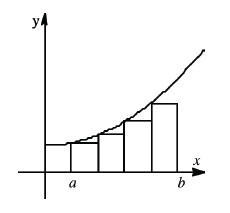Midpoint Rectangles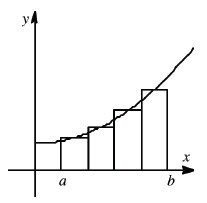Right Endpoint Rectangles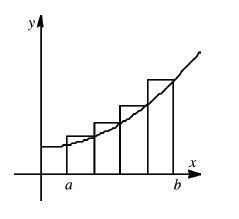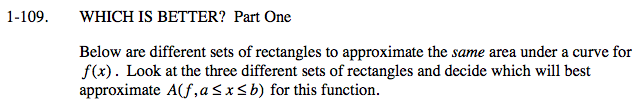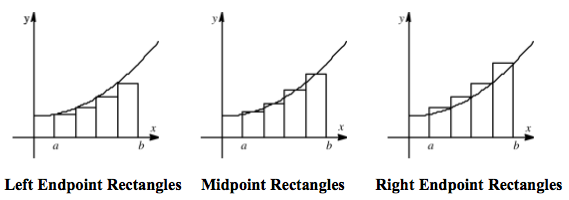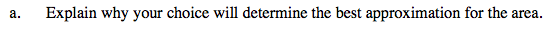Which graph is the closest to the exact area under the curve?

Midpoint Rectangles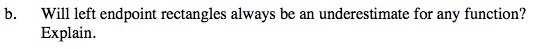Notice that the graphs in the diagrams above show an increasing function. That is, as x increases, the y-values increase. What if we graphed a decreasing function instead? Would left-endpoint rectangles be underestimates then?